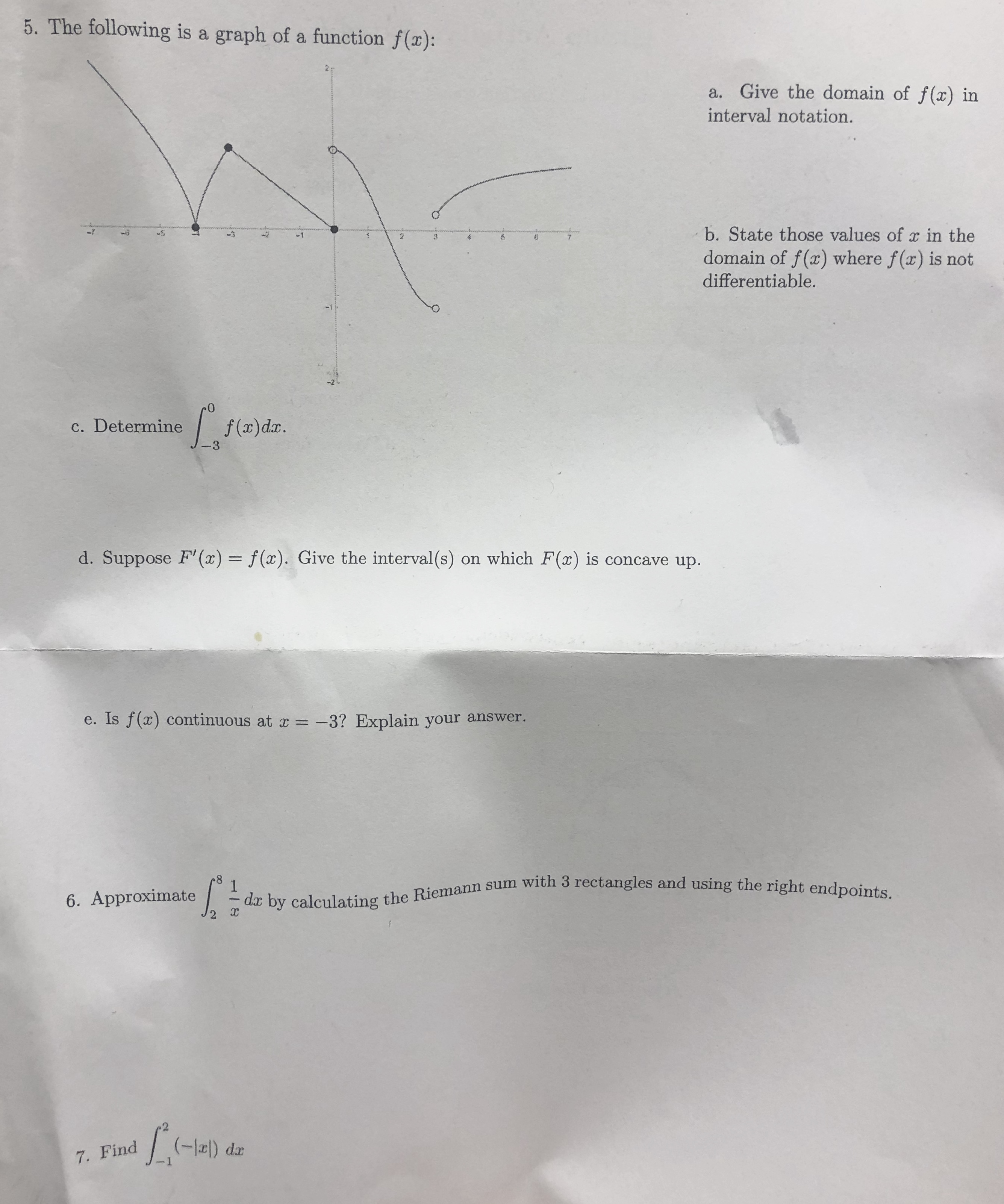# 5. The following is a graph of a function f(x):a. Give the domain of f(x) ininterval notation.b. State those values of r in the-337domain of f(x) where f(x) is notdifferentiable.efa)da.c. Determine-3d. Suppose F' (x) = f(x). Give the interval(s) on which F(x) is concave up.e. Is f(x) continuous at x-3? Explain your answer.- da by calculating the Riemann sum with 3 rectangles and using the right endpoints.16. Approximate7. Find

Question
1 views

Question 7help_outlineImage Transcriptionclose5. The following is a graph of a function f(x): a. Give the domain of f(x) in interval notation. b. State those values of r in the -3 3 7 domain of f(x) where f(x) is not differentiable. efa)da. c. Determine -3 d. Suppose F' (x) = f(x). Give the interval(s) on which F(x) is concave up. e. Is f(x) continuous at x -3? Explain your answer. - da by calculating the Riemann sum with 3 rectangles and using the right endpoints. 1 6. Approximate 7. Find fullscreen
check_circle

Step 1

We need to find the giv...

### Want to see the full answer?

See Solution

#### Want to see this answer and more?

Solutions are written by subject experts who are available 24/7. Questions are typically answered within 1 hour.*

See Solution
*Response times may vary by subject and question.
Tagged in

### Limits